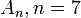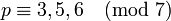# Alternating group:A7

View a complete list of particular groups (this is a very huge list!)[SHOW MORE]

## Definition

This group is defined as the alternating group of degree$7$, i.e., the alternating group on a set of size$7$. In other words, it is the subgroup of symmetric group:S7 comprising the even permutations.

## Arithmetic functions

### Basic arithmetic functions

Want to compare and contrast arithmetic function values with other groups of the same order? Check out groups of order 2520#Arithmetic functions
Function Value Similar groups Explanation
order (number of elements, equivalently, cardinality or size of underlying set) 2520 groups with same order As alternating group$A_n, n = 7$:$n!/2 = 7!/2$ which simplifies to$(7 \cdot 6 \cdot 5 \cdot 4 \cdot 3 \cdot 2 \cdot 1)/2 = 2520$
exponent of a group 420 groups with same order and exponent of a group | groups with same exponent of a group
derived length -- not a solvable group
nilpotency class -- not a nilpotent group
Frattini length 1 groups with same order and Frattini length | groups with same Frattini length Frattini-free group: intersection of all maximal subgroups is trivial
minimum size of generating set 2 groups with same order and minimum size of generating set | groups with same minimum size of generating set

### Arithmetic functions of a counting nature

Function Value Explanation
number of subgroups 3786 See subgroup structure of alternating group:A7, subgroup structure of alternating groups
number of conjugacy classes 9 See element structure of alternating group:A7, element structure of alternating groups
number of conjugacy classes of subgroups 40 See subgroup structure of alternating group:A7, subgroup structure of alternating groups

## Group properties

Property Satisfied Explanation Comment
Abelian group No$(1,2,3)$,$(1,2,3,4,5)$ don't commute$A_n$ is non-abelian,$n \ge 4$.
Nilpotent group No Centerless: The center is trivial$A_n$ is non-nilpotent,$n \ge 4$.
Metacyclic group No Simple and non-abelian$A_n$ is not metacyclic,$n \ge 4$.
Supersolvable group No Simple and non-abelian$A_n$ is not supersolvable,$n \ge 4$.
Solvable group No$A_n$ is not solvable,$n \ge 5$.
Simple non-abelian group Yes alternating groups are simple, projective special linear group is simple
T-group Yes Simple and non-abelian
Ambivalent group No Classification of ambivalent alternating groups
Rational-representation group No
Rational group No
Complete group No Conjugation by odd permutations in$S_6$ gives outer automorphisms.
N-group Yes See classification of alternating groups that are N-groups$A_n$ is a N-group only for$n \le 7$.

## Elements

Further information: element structure of alternating group:A7

## Subgroups

Further information: subgroup structure of alternating group:A7

### Quick summary

Item Value
Number of subgroups 3786
Compared with$A_n, n = 3,4,5,\dots$: 2, 10, 59, 501, 3786, 48337, ...
Number of conjugacy classes of subgroups 40
Compared with$A_n, n = 3,4,5,\dots$: 2, 5, 9, 22, 40, 137, ...
Number of automorphism classes of subgroups 37
Compared with$A_n, n = 3,4,5,\dots$: 2, 5, 9, 16, 37, 112, ...
Isomorphism classes of Sylow subgroups and the corresponding fusion systems 2-Sylow: dihedral group:D8 (order 8) as D8 in A7 (with its non-inner fusion system -- see fusion systems for dihedral group:D8). Sylow number is 315.
3-Sylow: elementary abelian group:E9 (order 9) as E9 in A7. Sylow number is 70.
5-Sylow: cyclic group:Z5 (order 5) as Z5 in A7. Sylow number is 126.
7-Sylow: cyclic group:Z7 (order 7) as Z7 in A7. Sylow number is 120.
Hall subgroups Other than the whole group, the trivial subgroup, and the Sylow subgroups, there are$\{ 2,3 \}$-Hall subgroups (of order 72) and$\{ 2,3,5 \}$-Hall subgroups (of order 360), the latter being A6 in A7. Note that the$\{ 2,3 \}$-Hall subgroups are not contained in$\{ 2,3,5 \}$-Hall subgroups.
maximal subgroups maximal subgroups have orders 72, 120, 168, 360.
normal subgroups only the whole group and the trivial subgroup, because the group is simple. See alternating groups are simple.
subgroups that are simple non-abelian groups (apart from the whole group itself) alternating group:A5 (order 60), projective special linear group:PSL(3,2) (order 168, the embedding arises via the natural permutation representation on the two-dimensional projective space over field:F2, which has size$2^2 + 2 + 1 = 7$), alternating group:A6 (order 360)

## Linear representation theory

Further information: linear representation theory of alternating group:A7

Item Value
degrees of irreducible representations over a splitting field 1,6,10,10,14,14,15,21,35
grouped form: 1 (1 time), 6 (1 time), 10 (2 times), 14 (2 times), 15 (1 time), 21 (1 time), 35 (1 time)
maximum: 35, lcm: 210, number: 9, sum of squares: 2520
Minimal splitting field, i.e., field of realization of all irreducible representations (characteristic zero)$\mathbb{Q}(\omega + \omega^2 + \omega^4)$ where$\omega$ is a primitive seventh root of unity. This is the same as$\mathbb{Q}(\sqrt{-7})$
Quadratic extension of$\mathbb{Q}$
Same as field generated by character values
Condition for a field of characteristic not 2,3,5, or 7, to be a splitting field -7 should be a square in the field.
Minimal splitting field, i.e., field of realization of all irreducible representations in prime characteristic$p \ne 2,3,5,7$ Case$p \equiv 1,2,4 \pmod 7$: prime field$\mathbb{F}_p$
Case$p \equiv 3,5,6 \pmod 7$: quadratic extension$\mathbb{F}_{p^2}$
Smallest size splitting field field:F11

## GAP implementation

Description Functions used
AlternatingGroup(7) AlternatingGroup
PerfectGroup(2520) or PerfectGroup(2520,1) PerfectGroup
SimpleGroup("Alt",7) SimpleGroup